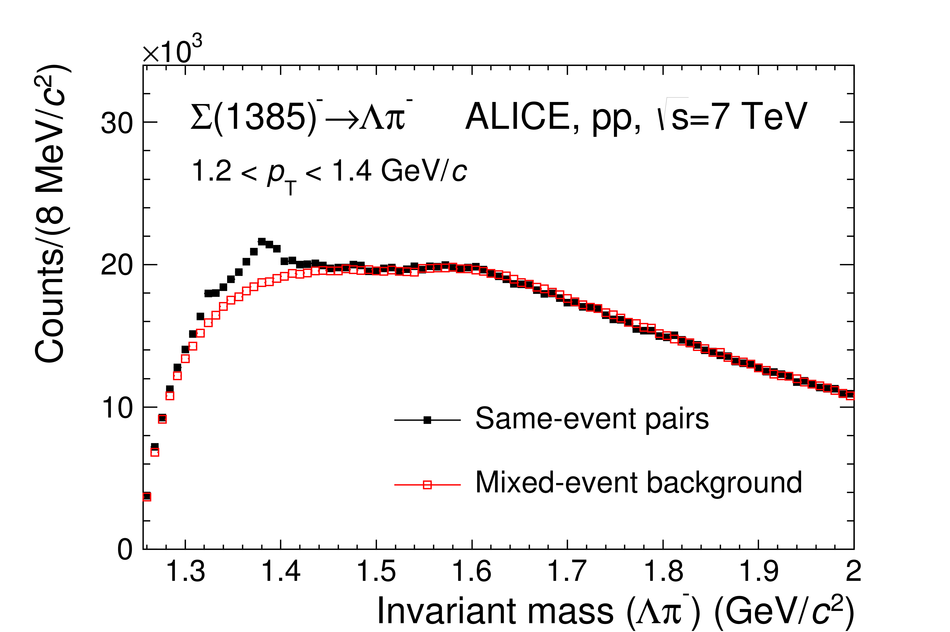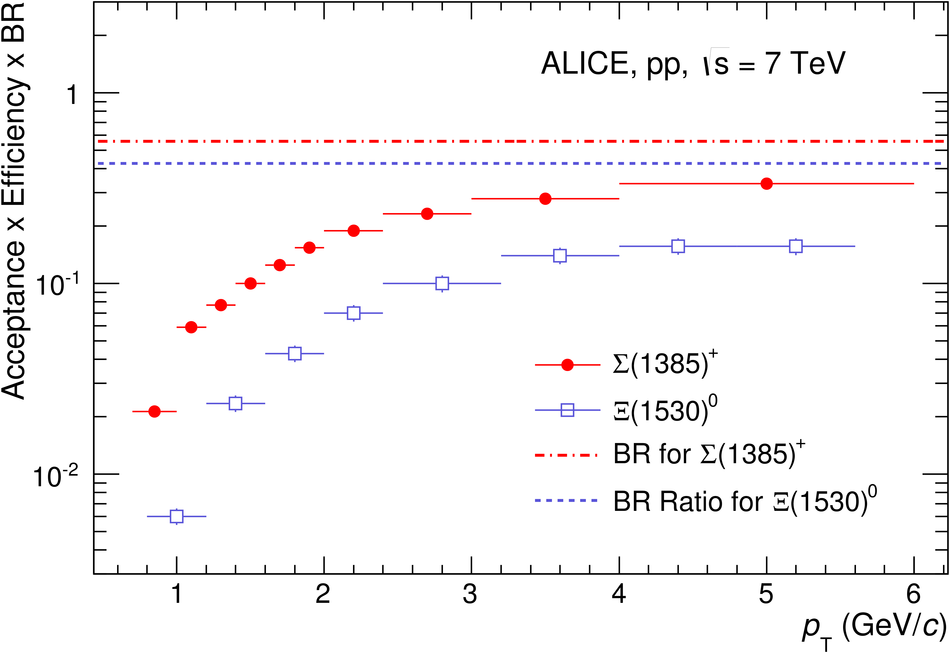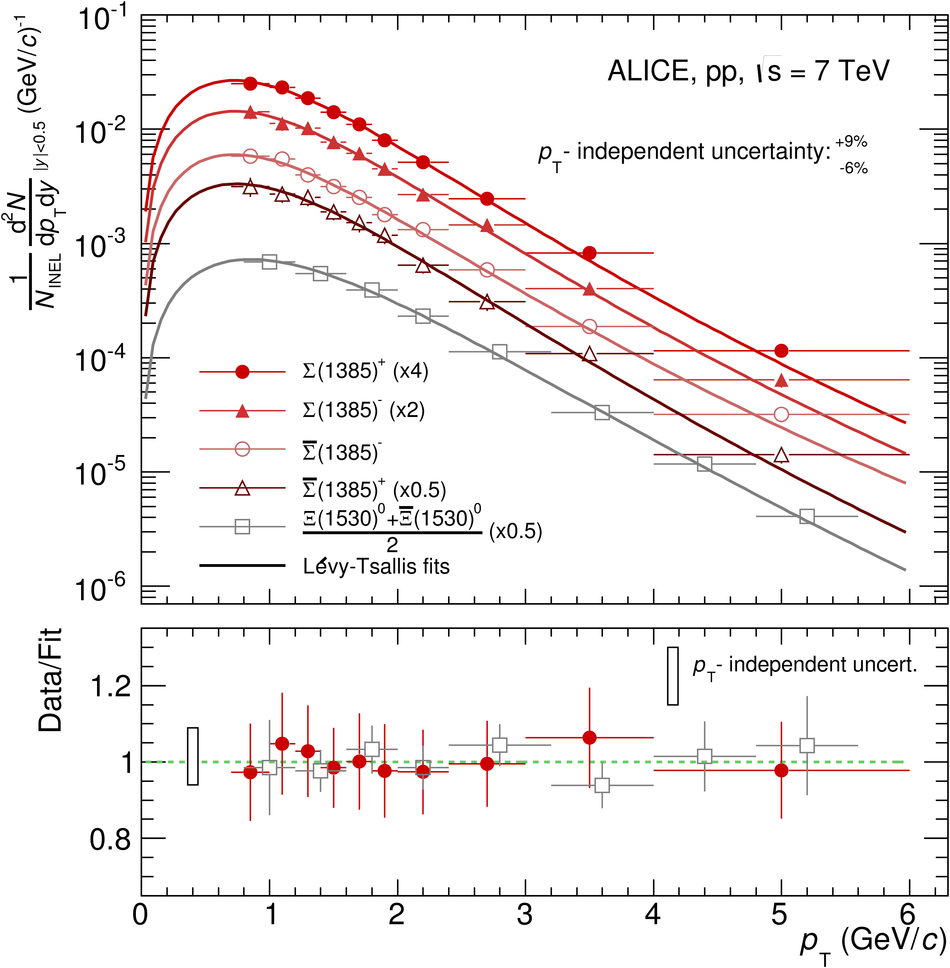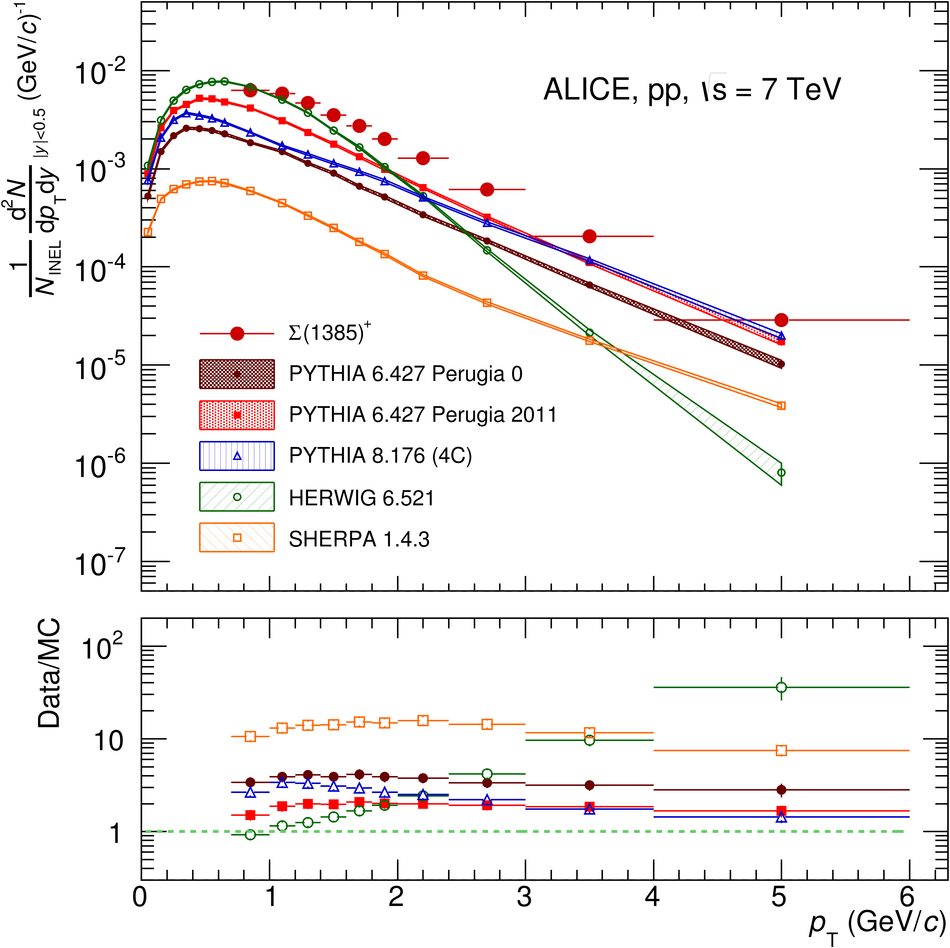# Production of $Σ(1385)^{\pm}$ and $Ξ(1530)^{0}$ in proton-proton collisions at $\sqrt{s}=$ 7 TeV

The production of the strange and double-strange baryon resonances ($\Sigma(1385)^{\pm}$, $\Xi(1530)^{0}$) has been measured at mid-rapidity ($\left | y \right |< 0.5$) in proton-proton collisions at $\sqrt{s}$ = 7 TeV with the ALICE detector at the LHC. Transverse momentum spectra for inelastic collisions are compared to QCD-inspired models, which in general underpredict the data. A search for the $\phi(1860)$ pentaquark, decaying in the $\Xi\pi$ channel, has been carried out but no evidence is seen.

Figures

## Figure 1

 (Top panel) The $\mathrm {\Lambda}$$\mathrm {\pi^+}invariant mass distribution in \left | y \right |$$<$0.5 for the transverse momentum bin 1.2$< $$p_{\rm T}$$<$1.4 GeV$\kern-0.15em /\kern-0.12em c$ in pp collisions at $\sqrt{s}$=7TeV The background shape estimated using pairs from different events (event-mixing) is shown as open red squares. The mixed-event background is normalized in the range 1.56$< M<$2.0 GeV$\kern-0.15em /\kern-0.12em c^2$, where $M$ is the $\mathrm {\Lambda}$$\mathrm {\pi^+}invariant mass (Bottom panel) The invariant mass distribution after mixed-event background subtraction for 1.2<$$p_{\rm T}$$<$1.4 GeV$\kern-0.15em /\kern-0.12em c$ The solid curve is the result of the combined fit (see text for details) and the dashed lines describes the residual background## Figure 2

 Same as Fig.1 but for $\mathrm {\Sigma}(1385)^{-}\longrightarrow\mathrm {\Lambda}+\mathrm {\pi^-}$ Note the peak at around the $\Xi(1321)^{-}$ mass, which is absent in Fig.1## Figure 3

 (Top panel) The $\mathrm {\Xi}^{-}\mathrm {\pi^+}$invariant mass distribution in $\left | y \right |$ < 0.5 for the transverse momentum bin 1.2< $p_{\rm T}$< 1.6 GeV$\kern-0.15em /\kern-0.12em c$ in pp collisions at $\sqrt{s}$=7TeV The background shape estimated using pairs from different events (event-mixing) is shown as open red squares. The mixed-event background is normalized in the range 1.49< M< 1.51 GeV$\kern-0.15em /\kern-0.12em c^2$ (Bottom panel) The invariant mass distribution after mixed-event background subtraction for 1.2< $p_{\rm T}$ < 1.6 GeV$\kern-0.15em /\kern-0.12em c$ The solid curve is the result of the combined fit and the dashed line describes the residual background.## Figure 4

 The product of acceptance, efficiency and branching ratio of $\mathrm {\Sigma}$(1385)$^+$ and $\mathrm {\Xi}$(1530)$^0$,obtained with PYTHIA 6.4 and GEANT3 ,as function of $p_{\rm T}$ in $\left | y \right |$< 0.5 Only statistical uncertainties are reported The dashed- and the dash-dotted lines indicate the overall branching ratio for the two reconstruction channels## Figure 5

 Inelastic baryon yields, ${\rm d}^2N/({\rm d}y {\rm d}p_{\rm T})$, per $p_{\rm T}$ interval per unit rapidity for $\mathrm {\Sigma}$(1385) and $\mathrm {\Xi (1530)^{0}}$. Statistical and systematic uncertainties are summed in quadrature, excluding the $p_{\rm T}$-independent uncertainties, which affect only the overall normalization of the spectra and are not considered in the fit Spectra are fitted with a Lévy-Tsallis function. The ratio data/fit is shown in the lower panel For the sake of visibility, only $\mathrm {\Sigma}$(1385)$^{+}$ is shown in the lower panel, but similar ratios have been obtained for the other three $\mathrm {\Sigma}$(1385) species. For the ratio, the integral of the fitting function in each corresponding $p_{\rm T}$ interval is considered Spectra points are represented at the centre of the $p_{\rm T}$ interval## Figure 6

 The transverse momentum spectrum of $\mathrm {\Sigma}$(1385)$^{+}$ is compared to standard tunes of PYTHIA 6 and PYTHIA 8, the latest release of HERWIG (6.521), and SHERPA release 1.4.6 The MC data are binned according to the data Spectra points are represented at the centre of the $p_{\rm T}$ interval The lower panel shows the ratio data/MC. $p_{\rm T}$-independent uncertainties are not shown## Figure 7

 Same as Fig. 6 but for $\mathrm {\Xi (1530)^{0}}$## Figure 8

 The mean $p_{\rm T}$ as function of the particle mass including $\mathrm {\Sigma}$(1385)$^+$, $\mathrm {\Xi (1530)^{0}}$ andother particles reconstructed in pp collisionsat $\sqrt{s}$ = 7 TeV and $\sqrt{s}$ = 0.9 TeV by the ALICE collaboration and at $\sqrt{s}$ = 0.2 TeV by the STAR collaboration The lower panel shows the ratio data/MC. Statistical and systematic uncertainties are shown separately (vertical solid lines and brackets, respectively)## Figure 9

 $\mathrm {\Xi}^{-}\mathrm {\pi^+}$ and $\mathrm {\Xi}^{-}\mathrm {\pi^+}$ invariant mass distributions The arrow and the shaded area indicate the region where the $\phi(1860)$ pentaquark isexpected and where the search was performed.